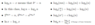# Big O/Little O, Big Ω, little ω

• Comp Sci
FritoTaco
Homework Statement:
For each pair of functions below, list which one(s) of the following are true:
##(1) f(n)=o(g(n)), \\
(2) f(n) = O(g(n)), \\
(3) f(n) = \Theta(g(n)), \\
(4) f(n) = \Omega(g(n)),\\
(5) f(n) = \omega(g(n)).##

##f(n)=\log(n+5), g(n)=\log(25n^2)##
Relevant Equations:
let ##f(n)## and ##g(n)## be functions mapping positive integers to positive real numbers.

##f(n)∈O(f(n))## if there exist positive constants ##c## and ##n_0## such that:
##f(n) \leq c \cdot g(n)## for all ##n > n_0##

##f(n)∈\Omega(g(n))## if there exist positive constants ##c## and ##n_0## such that:
##f(n) \geq c \cdot g(n)## for all ##n > n_0##

##f(n)∈ \theta(g(n))## iff ##f(n)∈ O(g(n))## and ##f(n) ∈ \Omega(g(n))##
##f(n) ∈ o(g(n))## iff ##f(n) ∈ O(g(n))## and ##f(n) ∉ \theta(g(n))##
##f(n) ∈ \omega(g(n))## iff ##f(n) ∈ \Omega(g(n))## and ##f(n) ∉ \theta(g(n))##
Just by looking at the two functions ##f(n)## and ##g(n)##, I can see that ##f(n)## is smaller
than ##g(n)## because of ##n^2##. To prove this. I attempted the following and I'm not sure
if I'm right.

##log(n+5) \leq c \cdot log(25n^2)## for all ##n \geq n_0##
##log(n+5) \leq c * log(25) + log(n^2)##
##log(n+5) \leq c * log(25) + 2 \cdot log(n)##

A quick note:
##log(n+5) \leq 2 \cdot log(n)##
##0 \leq log(25)##

##log(n+5) + 0 \leq 2 \cdot log(n) + log(25)##

##c = 2##
##n_0 = 1##

Therefore, ##log(n+5) ∈ O(log(25))##

That's my attempt to show it is big-O.
I'm not sure how to solve for ##\Omega##,
but my attempt is the same as the above.

##log(n+5) \geq log(25n^2)## for all ##n \geq n^0##

i can't simplify ##log(n+5)##

##c \cdot log(n+5) \geq log(25n^2)##

##c = 1##
so I pick a ##c## value and try to find a ##n_0##
such that ##1 \cdot log(n+5) \geq log(25n^2)##

I'll pick ##n_0 = 10##

##1 \cdot log(10+5) \geq log(25(10^2))##

this is false. But I don't understand how to conclude
that ##log(10+5) ∈ \Omega(log(25(10^2)))##
is false (which I assume it is false). Then I have no idea
how to begin to prove ##f(n) ∈ o(g(n))##. I've been given
an example like this:

##3n ∈ o(n^2)## because ##n < n^2## but,
##3n^2 ∉ o(n^2)## because ##n^2 \nless n^2##.
Do I have to do a new proof or can I just use ##n_0 = 1##
and plug it into ##f(n)=log(n+5))## and ##g(n)=log(25n^2)##?
Also, is my ##c## correct for the first proof?

#### Attachments

•logRules.PNG
7.1 KB · Views: 123
Last edited:

Mentor
##log(n+5) \leq c * log(25) + log(n^2)##
Here a factor c got lost.
##log(n+5) ∈ O(log(25))##
Huh? No. ##log(n+5) ∈ O(log(25n^2))## but that is coming from the n^2 term, the 25 doesn't play a role.

In the first formula for Ω there is a c missing.
##1 \cdot log(10+5) \geq log(25(10^2))## is false
That just tells you that n0=10 or c=1 (or both) are too small.

For large n the +5 becomes negligible (this a heuristic argument here). What does that mean for the ratio of the two functions? Does that give you an idea which c to study? That you can formalize then.

•FritoTaco